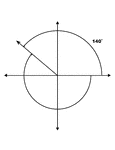### 140° and -220° Coterminal Angles

Illustration showing coterminal angles of 140° and -220°. Coterminal angles are angles drawn…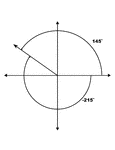### 145° and -215° Coterminal Angles

Illustration showing coterminal angles of 145° and -215°. Coterminal angles are angles drawn…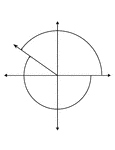### 145° and -215° Coterminal Angles

Illustration showing coterminal angles of 145° and -215°. Coterminal angles are angles drawn…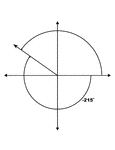### 145° and -215° Coterminal Angles

Illustration showing coterminal angles of 145° and -215°. Coterminal angles are angles drawn…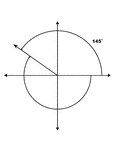### 145° and -215° Coterminal Angles

Illustration showing coterminal angles of 145° and -215°. Coterminal angles are angles drawn…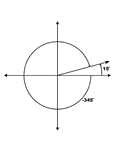### 15° and -345° Coterminal Angles

Illustration showing coterminal angles of 15° and -345°. Coterminal angles are angles drawn…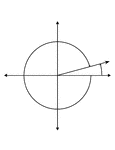### 15° and -345° Coterminal Angles

Illustration showing coterminal angles of 15° and -345°. Coterminal angles are angles drawn…### 15° and -345° Coterminal Angles

Illustration showing coterminal angles of 15° and -345°. Coterminal angles are angles drawn…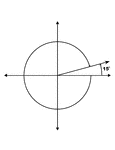### 15° and -345° Coterminal Angles

Illustration showing coterminal angles of 15° and -345°. Coterminal angles are angles drawn…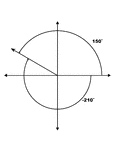### 150° and -210° Coterminal Angles

Illustration showing coterminal angles of 150° and -210°. Coterminal angles are angles drawn…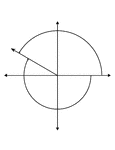### 150° and -210° Coterminal Angles

Illustration showing coterminal angles of 150° and -210°. Coterminal angles are angles drawn…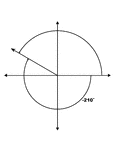### 150° and -210° Coterminal Angles

Illustration showing coterminal angles of 150° and -210°. Coterminal angles are angles drawn…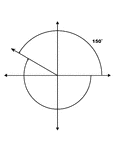### 150° and -210° Coterminal Angles

Illustration showing coterminal angles of 150° and -210°. Coterminal angles are angles drawn…### 155° and -205° Coterminal Angles

Illustration showing coterminal angles of 155° and -205°. Coterminal angles are angles drawn…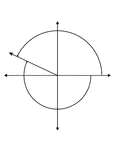### 155° and -205° Coterminal Angles

Illustration showing coterminal angles of 155° and -205°. Coterminal angles are angles drawn…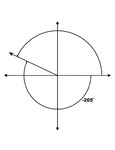### 155° and -205° Coterminal Angles

Illustration showing coterminal angles of 155° and -205°. Coterminal angles are angles drawn…### 155° and -205° Coterminal Angles

Illustration showing coterminal angles of 155° and -205°. Coterminal angles are angles drawn…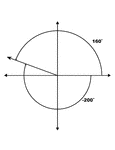### 160° and -200° Coterminal Angles

Illustration showing coterminal angles of 160° and -200°. Coterminal angles are angles drawn…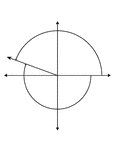### 160° and -200° Coterminal Angles

Illustration showing coterminal angles of 160° and -200°. Coterminal angles are angles drawn…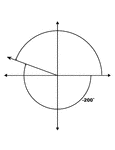### 160° and -200° Coterminal Angles

Illustration showing coterminal angles of 160° and -200°. Coterminal angles are angles drawn…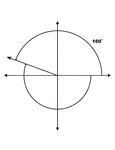### 160° and -200° Coterminal Angles

Illustration showing coterminal angles of 160° and -200°. Coterminal angles are angles drawn…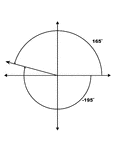### 165° and -195° Coterminal Angles

Illustration showing coterminal angles of 165° and -195°. Coterminal angles are angles drawn…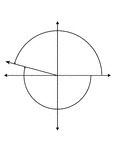### 165° and -195° Coterminal Angles

Illustration showing coterminal angles of 165° and -195°. Coterminal angles are angles drawn…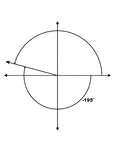### 165° and -195° Coterminal Angles

Illustration showing coterminal angles of 165° and -195°. Coterminal angles are angles drawn…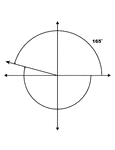### 165° and -195° Coterminal Angles

Illustration showing coterminal angles of 165° and -195°. Coterminal angles are angles drawn…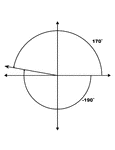### 170° and -190° Coterminal Angles

Illustration showing coterminal angles of 170° and -190°. Coterminal angles are angles drawn…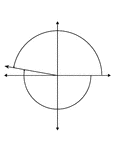### 170° and -190° Coterminal Angles

Illustration showing coterminal angles of 170° and -190°. Coterminal angles are angles drawn…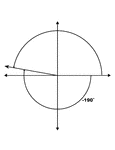### 170° and -190° Coterminal Angles

Illustration showing coterminal angles of 170° and -190°. Coterminal angles are angles drawn…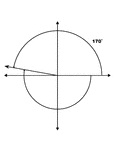### 170° and -190° Coterminal Angles

Illustration showing coterminal angles of 170° and -190°. Coterminal angles are angles drawn…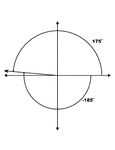### 175° and -185° Coterminal Angles

Illustration showing coterminal angles of 175° and -185°. Coterminal angles are angles drawn…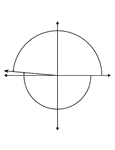### 175° and -185° Coterminal Angles

Illustration showing coterminal angles of 175° and -185°. Coterminal angles are angles drawn…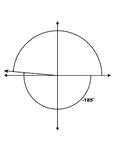### 175° and -185° Coterminal Angles

Illustration showing coterminal angles of 175° and -185°. Coterminal angles are angles drawn…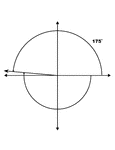### 175° and -185° Coterminal Angles

Illustration showing coterminal angles of 175° and -185°. Coterminal angles are angles drawn…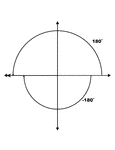### 180° and -180° Coterminal Angles

Illustration showing coterminal angles of 180° and -180°. Coterminal angles are angles drawn…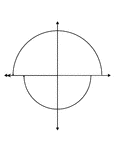### 180° and -180° Coterminal Angles

Illustration showing coterminal angles of 180° and -180°. Coterminal angles are angles drawn…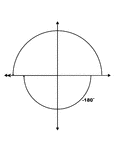### 180° and -180° Coterminal Angles

Illustration showing coterminal angles of 180° and -180°. Coterminal angles are angles drawn…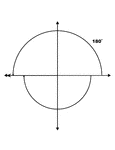### 180° and -180° Coterminal Angles

Illustration showing coterminal angles of 180° and -180°. Coterminal angles are angles drawn…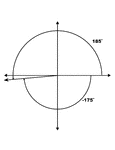### 185° and -175° Coterminal Angles

Illustration showing coterminal angles of 185° and -175°. Coterminal angles are angles drawn…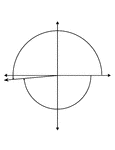### 185° and -175° Coterminal Angles

Illustration showing coterminal angles of 185° and -175°. Coterminal angles are angles drawn…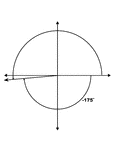### 185° and -175° Coterminal Angles

Illustration showing coterminal angles of 185° and -175°. Coterminal angles are angles drawn…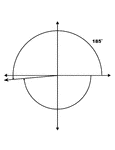### 185° and -175° Coterminal Angles

Illustration showing coterminal angles of 185° and -175°. Coterminal angles are angles drawn…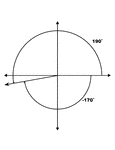### 190° and -170° Coterminal Angles

Illustration showing coterminal angles of 190° and -170°. Coterminal angles are angles drawn…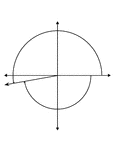### 190° and -170° Coterminal Angles

Illustration showing coterminal angles of 190° and -170°. Coterminal angles are angles drawn…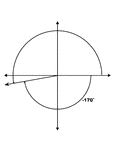### 190° and -170° Coterminal Angles

Illustration showing coterminal angles of 190° and -170°. Coterminal angles are angles drawn…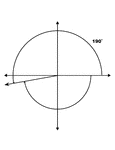### 190° and -170° Coterminal Angles

Illustration showing coterminal angles of 190° and -170°. Coterminal angles are angles drawn…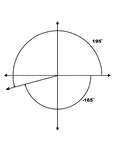### 195° and -165° Coterminal Angles

Illustration showing coterminal angles of 195° and -165°. Coterminal angles are angles drawn…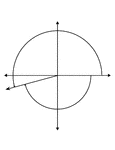### 195° and -165° Coterminal Angles

Illustration showing coterminal angles of 195° and -165°. Coterminal angles are angles drawn…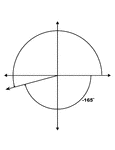### 195° and -165° Coterminal Angles

Illustration showing coterminal angles of 195° and -165°. Coterminal angles are angles drawn…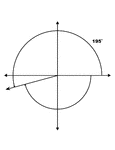### 195° and -165° Coterminal Angles

Illustration showing coterminal angles of 195° and -165°. Coterminal angles are angles drawn…### 20° and -340° Coterminal Angles

Illustration showing coterminal angles of 20° and -340°. Coterminal angles are angles drawn…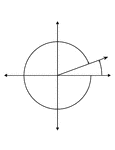### 20° and -340° Coterminal Angles

Illustration showing coterminal angles of 20° and -340°. Coterminal angles are angles drawn…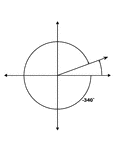### 20° and -340° Coterminal Angles

Illustration showing coterminal angles of 20° and -340°. Coterminal angles are angles drawn…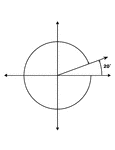### 20° and -340° Coterminal Angles

Illustration showing coterminal angles of 20° and -340°. Coterminal angles are angles drawn…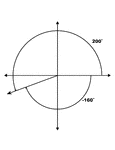### 200° and -160° Coterminal Angles

Illustration showing coterminal angles of 200° and -160°. Coterminal angles are angles drawn…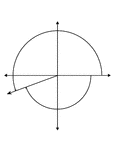### 200° and -160° Coterminal Angles

Illustration showing coterminal angles of 200° and -160°. Coterminal angles are angles drawn…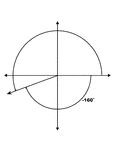### 200° and -160° Coterminal Angles

Illustration showing coterminal angles of 200° and -160°. Coterminal angles are angles drawn…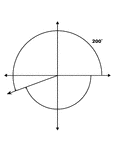### 200° and -160° Coterminal Angles

Illustration showing coterminal angles of 200° and -160°. Coterminal angles are angles drawn…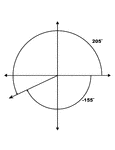### 205° and -155° Coterminal Angles

Illustration showing coterminal angles of 205° and -155°. Coterminal angles are angles drawn…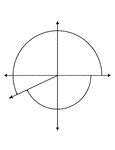### 205° and -155° Coterminal Angles

Illustration showing coterminal angles of 205° and -155°. Coterminal angles are angles drawn…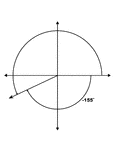### 205° and -155° Coterminal Angles

Illustration showing coterminal angles of 205° and -155°. Coterminal angles are angles drawn…